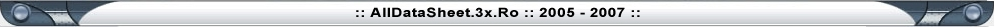Home
 Coils and transformers 1. Coils Coils are not that common components of electronic devices as resistors and capacitors are. They are encountered in various oscillators, radio-receivers, radio-emitters and similar devices containing oscillatory circuits. In amateur conditions, coil can be made by coiling one or more layers of isolated copper wire onto a cylindrical insulator body (PVC, cardboard, etc.) in a specified fashion. Factory made coils come in different shapes and sizes, but the common feature for all of them is an insulator body with coiled copper wire. Basic characteristic of every coil is its inductance. Inductance is measured in Henry (H), but more common are milihenry (mH) and microhenry (µH) as one Henry is quite high inductance value. As a reminder: 1H = 1000mH = 106 µH. Coil reactance is marked by XL, and can be calculated using the following formula: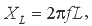where f represents the frequency of coil voltage in Hz and the L represents the coil inductance in H. For example, if f equals 684 kHz, while L=0.6 mH, coil reactance will be: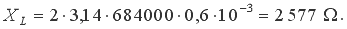The same coil would have three times higher reactive resistance at three times higher frequency and vice versa. As can be seen from the formula above, coil reactance is in direct proportion to voltage frequency, so that coils, as well as capacitors, are used in different circuits for filtering voltage of specified frequency. Note that coil reactance equals zero for DC, for f=0 in that case. Several coils are shown on the figures 3.1, 3.2, 3.3, and 3.4. The simplest form of coil is single-layer air core coil. It is made of cylindrical insulator body (PVC, cardboard, etc.) wrapped in isolated copper wire in specific pattern, as shown on the figure 3.1. On the figure 3.1a, curls have a certain amount of space left between them, while the common practice is to coil the wire with practically no space left between curls. To prevent coil unfolding, wire ends should be put through little holes as shown on the figure, but some sticky tape could also do the job.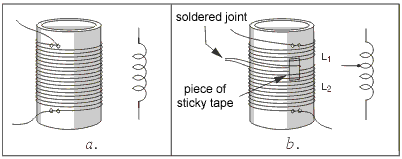Fig. 3.1: Single-layer coil w/o core: a. Regular, b. With an outconnector The figure 3.1b shows how the coil is made. For instance, if the coil totals 120 curls with an outconnector on the thirtieth curl, then there are two coils L1 with 30 curls and L2 with 90 curls one next to the other (or one over the other) on the same coil body. When the end of the first and the beginning of the second coil are soldered, we get the outconnector. Multilayered coil is shown on the figure 3.2a. The inner side of the plastic coil body is fashioned as a screwhole, so that the ferromagnetic coil core in shape of small screwbolt can fit in. Screwing the core moves it along the coil axis, and nearing it to the center of the coil increases the inductance. In this manner, fine inductance settings can be made.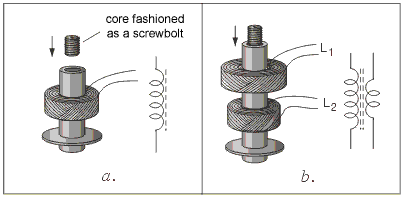Fig. 3.2: a. Multi-layered coil w core, b. Coupled coils The figure 3.2b shows the high-frequency transformer. As it can be seen, these are two coils coupled by magnetic induction on a shared body. In case when the coils are required to have exact specified inductance values, each coil has ferromagnetic core that can be moved along the coil axis. At very high frequencies (above 50mHz) required coil inductance value is relatively low, so these coils consist of merely few curls. These coils are made of thick, copper wire (approx. 1mm) with no coil body, as shown on the figure 3.3a. Their inductance can be adjusted by physical stretching or contracting.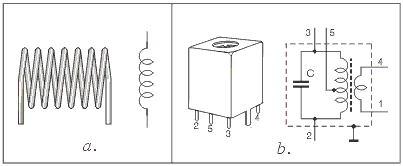Fig. 3.3: a. High frequency coil, b. Inter-frequency transformer The figure 3.3b shows the metal casing containing two bonded coils, with an electrical scheme on the right. The parallel connection of the first coil and the capacitor C forms an oscillatory circuit. The second coil is used for transferring the signal to the next block. This mechanism is used in receivers and similar devices. Metal casing serves as faradic cage, preventing the external magnetic influence and containing the internal magnetic field produced by the coil currents. In order to be used as a cage, metal casing has to be grounded. Coil in the "pot" casing made of ferromagnetic material is shown on the figure 3.4. These coils are used at lower frequencies (10kHz). Fine inductance adjustments can be made using the screw made of ferromagnetic material.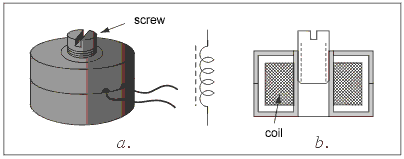Fig. 3.4: Coil in the "pot" casing: a. outlook, b. Symbol and a scheme Another kind of coils are the so called defusers featuring very high reactance at working frequency and very low resistance for DC. There are HF defusers  (used at high frequencies) and LF defusers (used at low frequencies). HF defusers look similar to the described coils. LF defusers are made with the cores identical to those used with network transformers. Symbol for HF defusers is the one used for the previously described coils, while the symbol for LF defusers looks like the one used for coils with core, with bold line or two thin lines instead of the broken line.  2. Transformers For electronic devices to function it is necessary to provide the DC power supply. Batteries and accumulators can fulfill the role, but much more efficient way is to use the converter. The basic component of the converter is the network transformer for transforming 220V to a certain lower value, say 12V. Network transformer has one primary coil which connects to the network voltage (220V) and one or several secondary coils for getting lower voltage values. Most commonly, cores are made of the so called E and I transformer sheet metal, but cores made of ferromagnetic tape are also used. There are also iron core transformers used at higher frequencies in converters. Various models of transformers are shown on the picture below.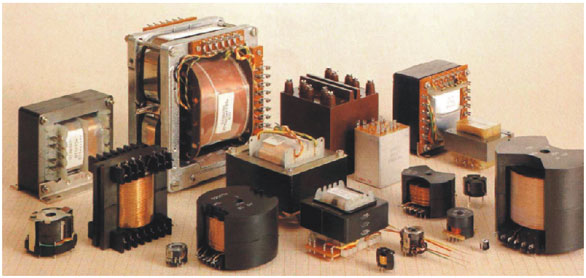Fig. 3.5: Various models of transformers Symbols of network transformers are shown on the figure 3.6; 3.6a and 3.6b are more accurate representations, while 3.6c and 3.6d are simpler to draw or print. Two vertical lines indicate that primary and secondary coils share the core made of transformer sheet metal.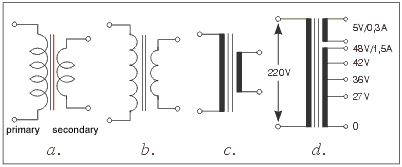Fig. 3.6: Transformer symbols With the transformer, manufacturers usually supply a scheme containing info on the primary and secondary coil, voltage and maximal currents. In case that this scheme is lacking, there is a simple method for determining which coil is the primary and which is the secondary: as primary coil consists of thinner wire and greater number of curls than the secondary, it has higher resistance value - the fact that can be easily tested by ohmmeter. The figure 3.6d shows the symbol for transformer with two independent secondary coils, one of them having three outconnectors. The secondary coil for getting 5V is made of thinner wire with maximal current 0.3A, while the other coil is made of thicker wire with maximal current 1.5A. Total voltage on the larger secondary coil is 48V, as shown on the figure  3.6d. Note that voltage values other than those marked on the scheme can be produced - for example, voltage between nodes marked as 27V and 36V equals 9V, voltage between nodes marked as 27V and 42V equals 15V, etc. 3. Working principles and basic characteristics of transformers As already stated, transformers consist of two coils, primary and the secondary (figure 3.7). When the voltage Up is brought to the primary coil (in our case it is network voltage, 220V) the AC current Ip flows through it. This current creates the alternate magnetic field which encompasses the secondary coil, inducing the voltage Us (24V in our example). Consumer is connected to the secondary coil - consumer is exemplified here with the resistor Rp (30Ω in our example). Of course, it is never a simple resistor but is some electronic device with an input resistance Rp. A simplest model would be an electric bulb working at 24V with electric power 19.2W. Most commonly it is a guiding part of the converter, consuming 0.8A current, etc.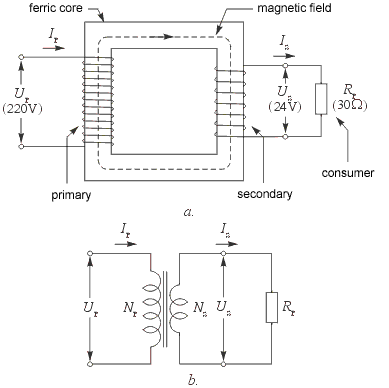Fig. 3.7: Transformer: a. Working principles, b. Symbol Transfer of electrical energy from the primary to the secondary coil is carried out via magnetic field. To prevent energy losses, it is necessary to assure that the whole magnetic field created by the primary coil encompasses the secondary. This is achieved by using the iron core, which has much lower magnetic resistance value than the air, thus containing almost entire magnetic field within the core. Basic characteristics of transformers are primary and secondary voltage, primary and secondary current (or power) and the efficiency. Primary voltage equals the network voltage. This value can be 220V or 110V, depending on the standards of the country. Secondary voltage is usually much lower, say 6V, 9V, 15V, 24V, etc, but can also be higher than 220V, depending on the transformer's purpose. Relation of the primary and the secondary voltage is given with the following formula: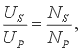where Ns and Np represent the number of curls of primary and secondary coil, respectively. For instance, if Ns equals 80 and Np equals 743, secondary voltage will be: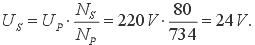Relation between the primary and the secondary current is described by the following formula: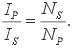For instance, if Rp equals 30Ω, than the secondary current equals Ip = Up/Rp = 24V/30Ω = 0.8A. If Ns equals 80 and Np equals 743, primary current will be: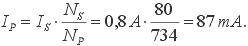Transformer power can be calculated by one of the following formulae: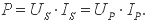In our example, the power equals: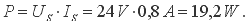Everything said up to this point relates to the ideal transformer. Clearly, there is no such thing as losses are inevitable. They are present due to the fact that the coil wire exhibits a certain resistance value, which makes the transformer warm up during the work, and the fact that the magnetic field created by the primary does not entirely encompass the secondary coil. This is why the electrical power of the secondary current has to be lower than the power of the primary current. Their ratio is called efficiency: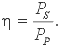For transformers with power measuring hundreds of Watts, efficiency is about µ=0.85, meaning that 85% of the electrical energy taken from the network gets to the consumer, while the 15% is lost due to previously mentioned factors in the form of heat. For example, if electrical power of the consumer equals Up*Ip = 30W, then the power which the transformer draws from the network equals: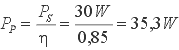To avoid any confusion here, bear in mind that manufacturers have already taken every measure in minimizing the losses of transformers and other electronic components and that, practically, this is the top possible efficiency for the present. When acquiring a transformer, you should only take care of the required voltage and the maximal current of the secondary coil. If the salesman cannot tell you the exact value of the current, he should be able to tell you the transformer's power. Dividing the values of power and the secondary voltage gets you the maximal current value for the consumer. Dividing the values of power and the primary voltage gets you the current that the transformer draws from network, which is important to know when buying the fuse. Anyhow, you should be able to calculate any value you might need using the appropriate formulae from above. 4. Practical examples with coils and transformers On the figure 2.6b coils, along with the capacitor, form two filters for conducting the currents to speakers. Coil and the capacitor C on the figure 2.6c form a parallel oscillatory circuit for filtering high-frequency radio signals, where the capacitor is used for tuning. Diode, 100pF capacitor and the headphones form a detector for filtering and reproducing audio signals.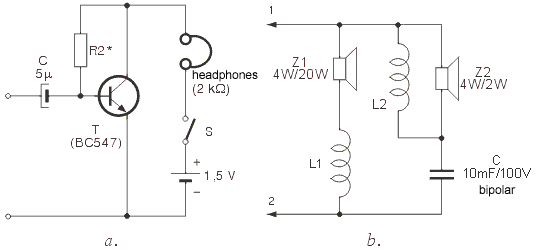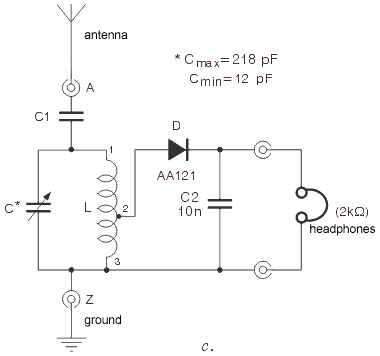Fig. 2.6: a. Amplifier with headphones, b. Electrical band-switch, c. Detector radio-receiver The most obvious application of transformers are converters, of course. One network transformer is shown on the figure 3.8 and is used for converting 220V voltage to 24V. Network voltage (220V) is brought to the primary coil using the on/off converter switch and the fuse that protects the converter from severe damage. It is very important that you bear in mind the fact that network voltage (220V) is very dangerous and to be careful when handling devices with network power supply. For practical realization of converter, one from the figure 3.8 or any other, the original power supply cable has to be used. There is no room for improvisation here, so don't experiment with ordinary isolated wires and such.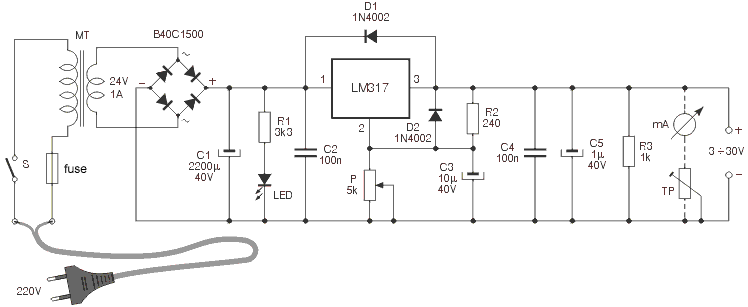Fig. 3.8: Stabilized converter with circuit LM317 Input DC voltage can be adjusted via linear potentiometer P, in 3~30V range.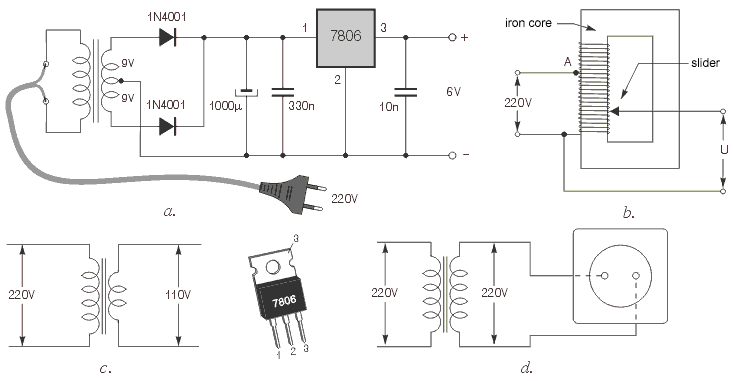Fig. 3.9: a. Stabilized converter with circuit 7806, b. auto-transformer, c. transformer for devices working at 110V, d. separating transformer The figure 3.9a shows a simple converter, using a network transformer with an outconnector on the middle of the secondary coil. This makes possible to use two diodes instead of the bridge from the figure 3.8. Special kind of transformers, mainly used in laboratories, are the auto-transformers. The scheme of an auto-transformer is given on the figure 3.9b. It features only one coil, coiled on the iron core used with regular transformers. Isolation is taken off from this coil's exterior so that the sliding contact could be attached. When the slider is in its lowest position, voltage equals zero. Moving the slider upwards raises the voltage U, reaching 220V in the node A. Further moving the slider increases the voltage U over 220V. Transformer from the figure 3.9c with secondary voltage 110V is used for supplying the devices supposed to work with network voltage 110V, as standards differ in various countries.  When using the converter for this purpose, bear in mind that problems may occur if the network voltage frequency is 60Hz instead of 50Hz. As the final example, figure 3.9d represents a scheme of the so called detachment transformer. This transformer features the same number of curls on primary and secondary coils. Secondary voltage is same as the primary, 220V, but is completely isolated from the public network, minimizing the risks of electrical shock. As a result, person can stand on the wet floor, etc and to touch and operate any part of the secondary coil without risks, which is not the case with the power outlet.Web Counter by TrafficFile.com